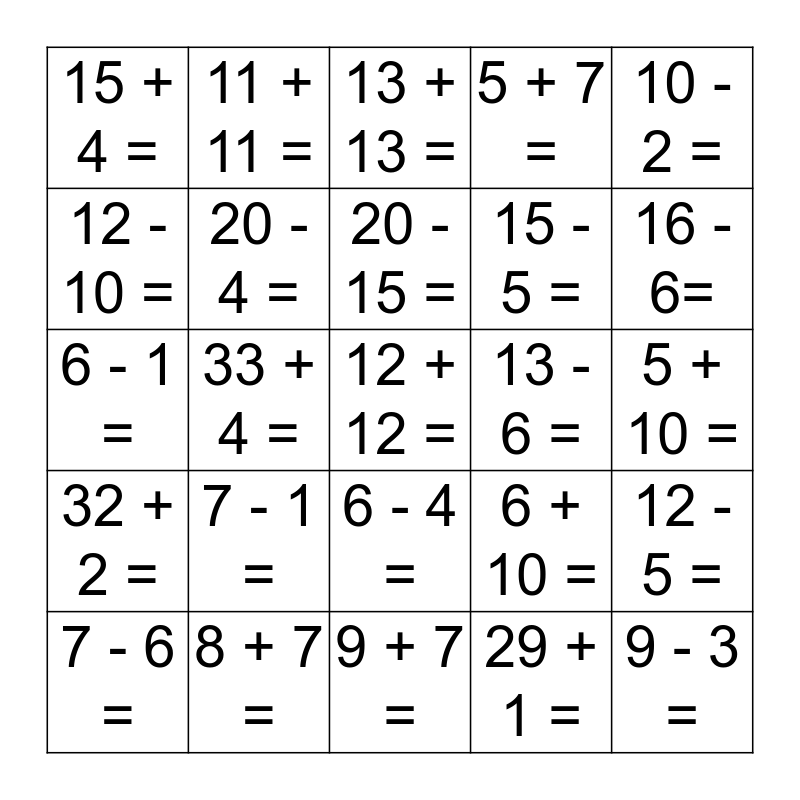# Math!This bingo card has 50 words: 9 + 9 =, 12 + 12 =, 6 + 6 =, 9 + 7 =, 7 - 1 =, 3 + 7 =, 11 + 11 =, 10 - 7 =, 12 - 10 =, 12 + 5 =, 20 - 4 =, 5 + 7 =, 12 - 5 =, 6 + 10 =, 7 + 9 =, 8 + 8 =, 20 - 15 =, 13 - 6 =, 8 + 4 =, 10 - 2 =, 33 + 4 =, 29 + 1 =, 15 + 4 =, 15 - 5 =, 4 + 4 =, 9 - 3 =, 16 - 6=, 8 + 1 =, 13 + 13 =, 6 - 4 =, 40 + 4 =, 9 + 9 =, 22 + 10 =, 6 - 2 =, 8 + 7 =, 3 - 3 =, 33 - 32 =, 8 - 4 =, 14 + 5 =, 5 + 10 =, 7 + 7 =, 15 - 6 =, 2 + 1 =, 9 + 5 =, 7 - 6 =, 6 - 1 =, 8 - 3 =, 4 + 4=, 32 + 2 = and 1 + 2 =.

⚠ This card has duplicate items: 9 + 9 = (2)

## Play Online